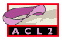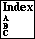## PROOF-OF-WELL-FOUNDEDNESS

a proof that `o<` is well-founded on `o-p`s
```Major Section:  MISCELLANEOUS
```

The soundness of ACL2 rests in part on the well-foundedness of `o<` on `o-p`s. This can be taken as obvious if one is willing to grant that those concepts are simply encodings of the standard mathematical notions of the ordinals below `epsilon-0` and its natural ordering relation. But it is possible to prove that `o<` is well-founded on `o-p`s without having to assert any connection to the ordinals and that is what we do here. The book `books/ordinals/proof-of-well-foundedness` carries out the proof outlined below in ACL2, using only that the natural numbers are well-founded.

Before outlining the above mentioned proof, we note that in the analogous documentation page of ACL2 Version_2.7, there is a proof of the well-foundedness of `e0-ord-<` on `e0-ordinalp`s, the less-than relation and recognizer for the old ordinals (that is, for the ordinals appearing in ACL2 up through that version). Manolios and Vroon have given a proof in ACL2 Version_2.7 that the current ordinals (based on `o<` and `o-p`) are order-isomorphic to the old ordinals (based on `e0-ord-<` and `e0-ordinalp`). Their proof establishes that switching from the old ordinals to the current ordinals preserves the soundness of ACL2. For details see their paper:

```Manolios, Panagiotis & Vroon, Daron.
Ordinal arithmetic in ACL2.
Kaufmann, Matt, & Moore, J Strother (eds).
Fourth International Workshop on the ACL2 Theorem
Prover and Its Applications (ACL2-2003),
July, 2003.
See URL `http://www.cs.utexas.edu/users/moore/acl2/workshop-2003/`.
```

We now give an outline of the above mentioned proof of well-foundedness. We first observe three facts about `o<` on ordinals that have been proved by ACL2 using only structural induction on lists. These theorems can be proved by hand.

```(defthm transitivity-of-o<
(implies (and (o< x y)
(o< y z))
(o< x z))
:rule-classes nil)

(defthm non-circularity-of-o<
(implies (o< x y)
(not (o< y x)))
:rule-classes nil)

(defthm trichotomy-of-o<
(implies (and (o-p x)
(o-p y))
(or (equal x y)
(o< x y)
(o< y x)))
:rule-classes nil)
```
These three properties establish that `o<` orders the `o-p`s. To put such a statement in the most standard mathematical nomenclature, we can define the macro:
```(defmacro o<= (x y)
`(not (o< ,y ,x)))
```
and then establish that `o<=` is a relation that is a simple, complete (i.e., total) order on ordinals by the following three lemmas, which have been proved:
```(defthm antisymmetry-of-o<=
(implies (and (o-p x)
(o-p y)
(o<= x y)
(o<= y x))
(equal x y))
:rule-classes nil
:hints (("Goal" :use non-circularity-of-o<)))

(defthm transitivity-of-o<=
(implies (and (o-p x)
(o-p y)
(o<= x y)
(o<= y z))
(o<= x z))
:rule-classes nil
:hints (("Goal" :use transitivity-of-o<)))

(defthm trichotomy-of-o<=
(implies (and (o-p x)
(o-p y))
(or (o<= x y)
(o<= y x)))
:rule-classes nil
:hints (("Goal" :use trichotomy-of-o<)))
```
Crucially important to the proof of the well-foundedness of `o<` on `o-p`s is the concept of ordinal-depth, abbreviated `od`:
```(defun od (l)
(if (o-finp l)
0
(1+ (od (o-first-expt l)))))
```
If the `od` of an `o-p` `x` is smaller than that of an `o-p` `y`, then `x` is `o<` `y`:
```(defun od-1 (x y)
(if (o-finp x)
(list x y)
(od-1 (o-first-expt x) (o-first-expt y))))

(defthm od-implies-ordlessp
(implies (and (o-p x)
(< (od x) (od y)))
(o< x y))
:hints (("Goal"
:induct (od-1 x y))))
```
Remark. A consequence of this lemma is the fact that if `s = s(1)`, `s(2)`, ... is an infinite, `o<` descending sequence of `o-p`s, then `od(s(1))`, `od(s(2))`, ... is a ``weakly'' descending sequence of non-negative integers: `od(s(i))` is greater than or equal to `od(s(i+1))`.

Lemma Main. For each non-negative integer `n`, `o<` well-orders the set of `o-p`s with `od` less than or equal to `n` .

``` Base Case.  n = 0.  The o-ps with 0 od are the non-negative
integers.  On the non-negative integers, o< is the same as <.

Induction Step.  n > 0.  We assume that o< well-orders the
o-ps with od less than n.

If o< does not well-order the o-ps with od less than or equal to n,
consider, D, the set of infinite, o< descending sequences of o-ps of od
less than or equal to n.  The first element of a sequence in D has od n.
Therefore, the o-first-expt of the first element of a sequence in D has od
n-1.  Since o<, by IH, well-orders the o-ps with od less than n, the set
of o-first-expts of first elements of the sequences in D has a minimal
element, which we denote by B and which has od of n-1.

Let k be the minimum integer such that for some infinite, o< descending
sequence s of o-ps with od less than or equal to n, the first element of s
has an o-first-expt of B and an o-first-coeff of k.  Notice that k is
positive.

Having fixed B and k, let s = s(1), s(2), ... be an infinite, o<
descending sequence of o-ps with od less than or equal to n such that s(1)
has a o-first-expt of B and an o-first-coeff of k.

We show that each s(i) has a o-first-expt of B and an o-first-coeff of
k. For suppose that s(j) is the first member of s either with o-first-expt
B and o-first-coeff m (m neq k) or with o-first-expt B' and o-first-coeff
B' (B' neq B). If (o-first-expt s(j)) = B', then B' has od n-1 (otherwise,
by IH, s would not be infinite) and B' is o< B, contradicting the
minimality of B. If 0 < m < k, then the fact that the sequence beginning
at s(j) is infinitely descending contradicts the minimality of k. If m >
k, then s(j) is greater than its predecessor; but this contradicts the
fact that s is descending.

Thus, by the definition of o<, for s to be a decreasing sequence of o-ps,
(o-rst s(1)), (o-rst s(2)), ... must be a decreasing sequence. We end by
showing this cannot be the case. Let t = t(1), t(2), ... be an infinite
sequence of o-ps such that t(i) = (o-rst s(i)). Then t is infinitely
descending. Furthermore, t(1) begins with an o-p B' that is o< B. Since t
is in D, t(1) has od n, therefore, B' has od n-1. But this contradicts the
minimality of B. Q.E.D.
```
Theorem. `o<` well-orders the `o-p`s. Proof. Every infinite,` o<` descending sequence of `o-p`s has the property that each member has `od` less than or equal to the `od`, `n`, of the first member of the sequence. This contradicts Lemma Main. Q.E.D.